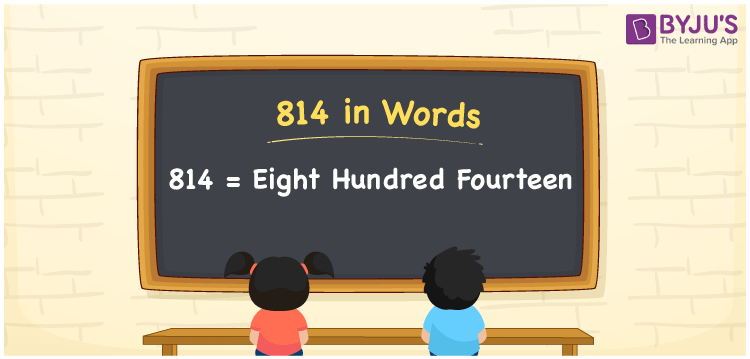# 814 in words

814 in words is written as Eight Hundred and Fourteen. 814 represents the count or value. The article on Counting Numbers can give you an idea about count or counting. The number 814 is a 3 digit number that is used in expressions related to money, days, distance, length, weight and so on. Let us consider an example for 814. “The city central library has a collection of Eight Hundred and Fourteen books.” Another example is “There are Eight Hundred and Fourteen flats in this society”

 814 in words Eight Hundred and Fourteen Eight Hundred and Fourteen in Numbers 814

## 814 in English Words## How to Write 814 in Words?

We can convert 814 to words using a place value chart. The number 814 has 3 digits, so let’s make a chart that shows the place value up to 3 digits.

 Hundreds Tens Ones 8 1 4

Thus, we can write the expanded form as:

8 × Hundred + 1 × Ten + 4 × One

= 8 × 100 + 1 × 10 + 4 × 1

= 814

= Eight Hundred and Fourteen.

814 is the natural number that is succeeded by 813 and preceded by 815.

814 in words – Eight Hundred and Fourteen.

Is 814 an odd number? – No.

Is 814 an even number? – Yes.

Is 814 a perfect square number? – No.

Is 814 a perfect cube number? – No.

Is 814 a prime number? – No.

Is 814 a composite number? – Yes.

## Solved Example

1. Write the number 814 in expanded form

Solution: 8 × 100 + 1 × 10 + 4 × 1

We can write 814 = 800 + 10 + 4

= 8 × 100 + 1 × 10 + 4 × 1.

## Frequently Asked Questions on 814 in words

Q1

### How to write the number 814 in words?

814 in words is written as Eight Hundred and Fourteen.
Q2

### State True or False. 814 is divisible by 2?

True. 814 is divisible by 2.
Q3

### Is 814 a perfect cube number?

No. 814 is not a perfect cube number.